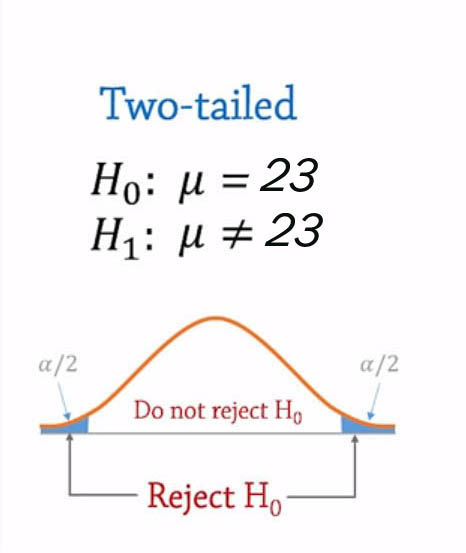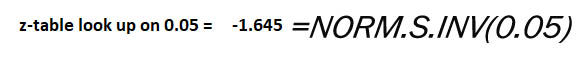top of page
Search
• Marek Vavrovic

# Hypothesis testing, p-value, test statistic, z-score.

Updated: Jun 8, 2020

• Hypothesis testing is just a method for testing a claim or hypothesis about a parameter in a population [mean, proportion]. In hypothesis testing we study the sample. Results of the sample are generalized to entire population.

• The “Null Hypothesis” denoted as H0 , this means testing a claim that already has some established parameters.

• The “Alternative Hypothesis” is denoted as H1, this is known as the research hypothesis. It involves the claim to be tested.

• Four steps of hypothesis testing are: (I) We state the Hypothesis (II) Set the criteria for a decision (III) Compute the test statistic (IV) Make a decision

Hypothesis testing is just a method for testing a claim or hypothesis about a parameter in a population, using data measured in a sample. The goal of hypothesis testing is to determine the likelihood that a population parameter, such as the mean (µ), is likely to be true.

The “Null Hypothesis” denoted as H0, this means testing a claim that already has some established parameters. The null hypothesis is always the accepted fact. It is a starting point. We test whether the value stated in the null hypothesis is likely to be true.

The “Alternative Hypothesis” is denoted as H1, this is known as the research hypothesis. It involves the claim to be tested. An alternative hypothesis (H0) is a statement that directly contradicts a null hypothesis by stating that that the actual value of a population parameter is less than, greater than, or not equal to the value stated in the null hypothesis. The alternate hypothesis is formulated depending on whether a one-tail or two-tail test is required:

Four steps of hypothesis testing:

Step 1: Define the Null Hypothesis. We start by assuming that the hypothesis or claim we are testing is true.

Step 2: Set the criteria for a decision. To set the criteria for a decision, we state the level of significance for a test. Level of significance refers to a criterion of judgment upon which a decision is made regarding the value stated in a null hypothesis. The likelihood or level of significance (α) is typically set at 5% [α=0.05] in behavioural research studies.

Step 3: Compute the test statistic. The test statistic is a mathematical formula that allows researchers to determine the likelihood of obtaining sample outcomes if the null hypothesis were true. The value of the test statistic is used to decide regarding the null hypothesis.

Step 4: Make a decision. We use the value of the test statistic to decide about the null hypothesis. The decision is based on the probability of obtaining a sample mean, given that the value stated in the null hypothesis is true. There are two decisions a researcher can make; either reject the null hypothesis or fail to reject the null hypothesis. We cannot prove the null hypothesis.

Let us have a look on couple of examples to understand this topic better.

Exercise #1 – Mean, Two sample mean test using z-score

A company is looking to improve their web reporting performance because the load time takes too long. Currently pages have a mean load time of µ0=4.25 seconds, with a standard deviation of σ=0.75 seconds. They hire a consulting firm to fix the code and improve report load times. Management wants a 99% confidence level α=0.01. After all has been fixed a sample of n=55 report pages has a new mean load time of 2.88.

Question is: are these results statistically faster than before?

1. Define null hypothesis

H0: µ0 >= 4.25

2. Define alternative hypothesis

H1: µ1 < 4.25

3. Set a level of significance

α=0.01

4. Determine the test type

We determine the test type based on that what the alternative hypothesis say.

It says LESS THAN…A: Testing solution - mean, traditional method

σ: population standard deviationcritical value in excel:Result: Reject the Null Hypothesis H0: µ0 >= 4.25

the test statistics falls INSIDE THE REJECTION REGION

-13.5469 < - 2.325

We can say that the new report pages are statistically faster.

B: Testing solution , P-value method

1. calculate p-valueresult: 5.62826E-41 < 0.0119

If p-value is low [less than 0.0119] the null hypothesis must go

REJECT NULL HYPOTHESIS

Exercise #2 – Proportion, using z-score

A music company surveys 350 of their customers and finds that 57% of the sample are under 21 years old. Is it fair to say that most of the company’s customers are teenagers?

We can translate MOST OF THE COMPANY’S CUSTOMERS to MORE THAN 50%.

1. Set the null hypothesis: H0: P<=0.50.

Null hypothesis says that most of the population are not mostly teenagers.

2. Set alternative hypothesis: H1: P>0.50

Alternative hypothesis says the opposite of null hypothesis.

3. Calculate the test statistics:

n=350 p=0.574. Set a significance level: α=0.05

5. Decide what type of tail is involved based on what alternative hypothesis says:

H1: P>0.50 [choose a right-tail test]6. Look up the critical value.

I'm going to do it Excel. You must convert it to positive because it is on the right side.The critacal value Z=1.645Based on the sample, we REJECT THE NULL HYPOTHSIS, and support the claim that most customers are teenagers.

the test statistic falls INSIDE THE REJECTION REGION

TEST STATISTIC > CRITICAL VALUE

2.59 > 1.645

EXAMPLE #3: Hypothesis z-test for One Sample Mean in Excel

Is there enough evidence at 90% confidence interval (α=0.10) to conclude that the population mean age differs significantly from 23 based on the following sample?

25 30 23 21 24 22 24 25 22 21 22 18 20 24 24 22 23 19 21 20 21 21 19 21 19 24 20 20 20 23 22 23 19 22 19

1. Set the null hypothesis: Ho: µ = 23

2. Set alternative hypothesis: Ha: µ=/= 23

3. Calculate the variance: F-Test Two sample for variances

I'm using a dummy variable because I have just one sample.You can also calculate variance using excel function VAR.S(), or Descriptive Statistics from Data Analysis tool pack.4. Calculate Z-Test: two-samples for mean: z-Test: Two Sample for Means needs sample variance to calculate the output.5. Decide what type of tail is involved6. Define rejection region

Reject Ho IF z < -1.645 or z > 1.645z-score is OUTSIDE THE CRITAL VALUES -1.645 & + 1.645. We reject the null hypothesis.

CONCLUSION: There IS NOT enough evidence at 90% confidence interval (α=0.10) to conclude that the population mean age differs significantly from 23 based on the sample.

P(Z<=z) two-tail < Confidence interval

0.00278 < 0.10

We can only reject the null hypothesis when the p-value IS LESS THAN the confidence interval P<=α

EXAMPLE #4: Hypothesis z-test for Two Sample Mean, no data, traditional method.

In recent years, the mean age of all college students in city XY has been 23. A random sample of 45 students revealed a mean age of 23.9. Suppose their ages are normally distributed with a population standard deviation of σ=2.4. Can we infer at α=0.05 that the population mean age has change?

1. Set the null hypothesis: Ho: µ = 23

2. Set alternative hypothesis: Ha: µ=/= 23

3. Set a level of significance: α=0.05

4. Determine the test type:

We determine the test type based on that what the alternative hypothesis say.

It says NOT EQUAL…Result: Reject the Null Hypothesis Ho: µ = 23

the test statistics falls INSIDE THE REJECTION REGION

Rejection region at α=0.05

Reject Ho if z < -1.645 or z > 1.645

Test Statistic: 2.516We can only reject the null hypothesis when the p-value IS LESS THAN the confidence interval P<=α 0.0168 < 0.05